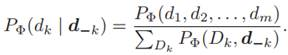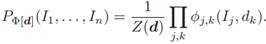# Consider the setting of example 12.18, where we assume that all grades are observed but none of…

Consider the setting of example 12.18, where we assume that all grades are observed but none of the Ij or Dk variables are observed. Show how you would use the set of collapsed samples generated in this example to compute the expected value of the number of smart students (i1) who got a grade of a C (g3) in an easy class (d0).

Example 12.18

Consider the Markov network defined by the Bayesian network of example 12.11, reduced over the evidence G = g. This Markov network is a bipartite graph, where we have two sets of variables I, D such that the only edges in the network are of the form Ij, Dk. Let φj,k(Ij , Dk) be the factor associated with each such edge. (For simplicity of notation, we assume that there is a factor for every such edge; the factors corresponding to grades that were not in the model will be vacuous.) We can now apply collapsed Gibbs sampling, selecting to sample the variables D and maintain a distribution over I; this choice will (in most cases) be better, since there are usually more students than courses, and it is generally better to sample in a lower-dimensional space. Thus, our Markov chain needs to be able to sample Dk from PΦ(Dk | d−k):The expression in the numerator, and each term in the sum in the denominator, is the probability of a full assignment to D. This type of expression can be computed as the partition function of the reduced Markov network that we obtain by setting D = d:Each of the factors in the product is a singleton over an individual Ij. Thus, we can compute the partition function or marginals of this distribution in linear time. In particular, we can easily compute the Gibbs transition probability. Using the same analysis, we can easily compute an expression for PΦ[d](I) in closed form, as a product of individual factors over the Ij’s. Thus, we can also easily compute expectations of any function over these variables, such as the expected value of the number of smart students who got a grade of a C in an easy class.